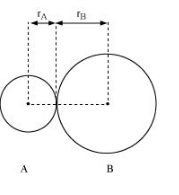# Define the bond length.

Question:

Define the bond length.

Solution:

Bond length is defined as the equilibrium distance between the nuclei of two bonded atoms in a molecule.

Bond lengths are expressed in terms of Angstrom $\left(10^{-10} \mathrm{~m}\right)$ or picometer

$\left(10^{-12} \mathrm{~m}\right)$ and are measured by spectroscopic $X$-ray diffractions and electron-diffraction techniques.

In an ionic compound, the bond length is the sum of the ionic radii of the constituting atoms $\left(d=r_{+}+r_{-}\right)$. In a covalent compound, it is the sum of their covalent radii ( $d=r_{A}+r_{B}$ ).